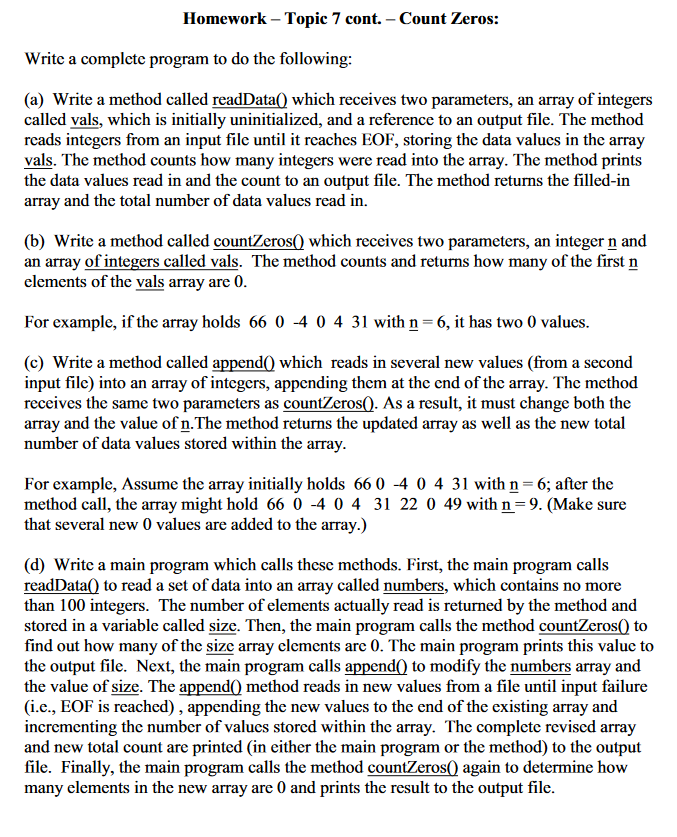WZMLBS The Asker · Computer Science

my code:

import java.io.*;
import java.util.Scanner;

public class homework7b {

public static int readData(int[] numbers, Scanner inputFile) throws IOException {

//Variable to count numbers
int n=0;
int count=0;
int [] array = new int;

//Create an output file object using the PrintWriter Class
PrintWriter outputFile = new PrintWriter("homework7.2output.txt");
//PrintWriter oututFile = new PrintWriter(System.out);

// read and count the number of marks
System.out.print("Enter the marks - end with EOF: ");

//Test for EOF
while (inputFile.hasNext())
{
numbers[n] = inputFile.nextInt();
count++;
n++;
}

for(n=0;n<count;n++) {
outputFile.print("\t"+ numbers[n]);
}
return count;
}

// countZeros() method
public static int countZeros(int array[],int len){
int count=0;
for(int n=0;n<len;n++)
{
if(array[n]==0)
{
count++;
}
}
return count;
}

//Append() method
public static int [] append(int array[],int len) throws IOException
{
Scanner input=new Scanner(new File("input2.txt"));
int new_length=0;
while(input.hasNext())
{
int num = input.nextInt();
new_length++;
}
int [] new_array=new int[len+new_length];
for(int n=0;n<len;n++)
{
new_array[n]=array[n];
}
input=new Scanner(new File("homework7dinput.txt"));
for(int n=0;n<new_length;n++)
{
new_array[len+n]=input.nextInt();
}
return new_array;
}

public static void main(String [] args) throws Exception {
int [] array=new int;

//Open the input file
File myFile = new File("homework7cinput.txt");

//Create a Scanner object to read the input file
Scanner inputFile = new Scanner(myFile);
//Scanner inputFile = new Scanner(System.in);

//Open the input file #2
File myFile1 = new File("homework7dinput.txt");

//Create a Scanner object to read the input file
Scanner inputFile1 = new Scanner(myFile1);
//Scanner inputFile = new Scanner(System.in);

//Create an output file object using the PrintWriter Class
PrintWriter outputFile = new PrintWriter("homework7.2output.txt");
//PrintWriter oututFile = new PrintWriter(System.out);

// read and count the number of marks
System.out.print("Enter the marks - end with EOF: ");

int len = readData (array,new Scanner(new File("homework7cinput.txt")));
print_array(array,len);
outputFile.println("Number of zeroes: "+ countZeros(array,len));
array = append(array,len);
print_array(array,array.length);
outputFile.println("Number of zeroes: "+countZeros(array,array.length));
}

//Method to print the array
public static void print_array(int array[],int len)throws IOException {
outputFile.print("Array: ");
for(int n=0;n<len;n++) {
outputFile.print(array[n]+" ");
}
outputFile.println();
}
}More